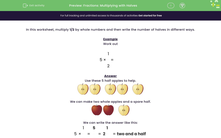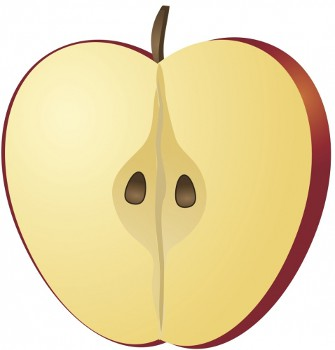# Fractions: Multiplying with Halves

In this worksheet, students multiply fractions.Key stage:  KS 2

Curriculum topic:   Number: Multiplication and Division

Curriculum subtopic:   Solve Multiplication/Division Problems with Fractions

Difficulty level:#### Worksheet Overview

In this worksheet, multiply 1/2 by whole numbers and then write the number of halves in different ways.

Example

Work out

5  ×
 1 2
=

Use these 5 half apples to help.We can make two whole apples and a spare half.We can write the answer like this:

5 ×
 1 2
=
 5 2
= 2
 1 2
= two and a half

### What is EdPlace?

We're your National Curriculum aligned online education content provider helping each child succeed in English, maths and science from year 1 to GCSE. With an EdPlace account you’ll be able to track and measure progress, helping each child achieve their best. We build confidence and attainment by personalising each child’s learning at a level that suits them.

Get started